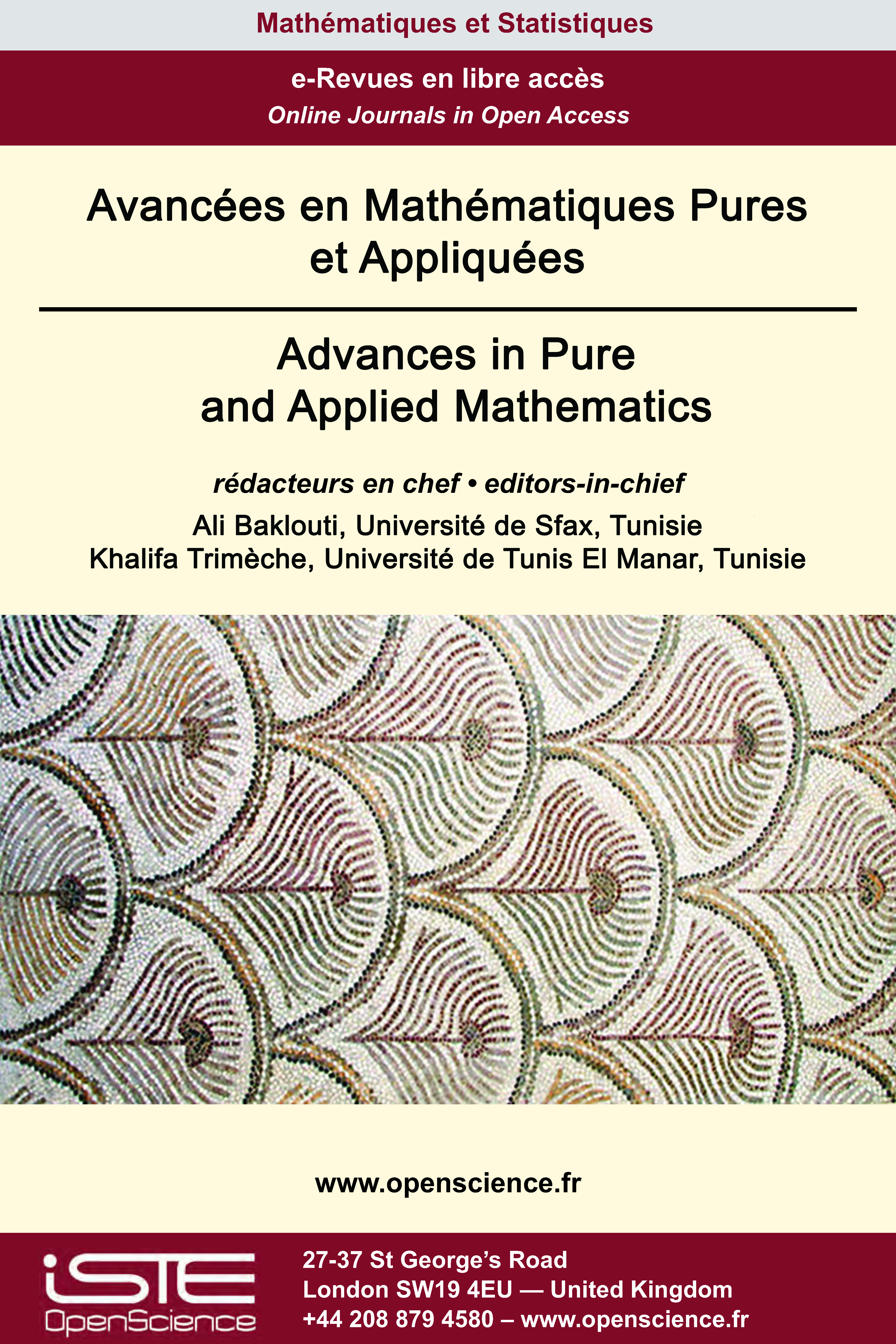Vol 13 - Issue 1 (January 2022)

List of Articles

Optimal control of a fractional diffusion Sturm-Liouville problem on a star graph

This paper is devoted to parabolic fractional boundary value problems involving fractional derivative of Sturm-Liouville type. We investigate the existence and uniqueness results on an open bounded real interval, prove the existence of solutions to a quadratic boundary optimal control problem and provide a characterization via optimality system. We then investigate the analogous problems for a parabolic fractional Sturm-Liouville problem on a star graph with mixed Dirichlet and Neumann boundary controls.

Complex symmetric weighted composition operators on weighted Hardy space

In this paper various conditions under which a weighted composition operator $W_{\psi,\phi}$ on the weighted Hardy space $H^2(\beta)$ becomes complex symmetric with respect to some special conjugation have been explored. We also investigate some important properties of the complex symmetric operator $W_{\psi,\phi}$ such as hermiticity and isometry.

Krasnoselskii-type fixed point theorem in ordered Banach spaces and application to integral equations

In this paper, we present a variant of Krasnoselskii’s fixed point theorem in the case of ordered Banach spaces, where the order is generated by a normal and minihedral cone. In such a structure, there is a possibility to give a new sence to the concept of contraction.

Terracini Loci and Homogeneous Spaces

We study the linear dependence of disjoint unions of double points of an integral and non-degenerate variety $X\subset ℙ^r$. Such sets are called Terracini loci. Our main results are for Segre-Veronese embeddings and a few other homogeneous spaces. To study the minimal number of such double points which are linearly dependent, it is useful to study the minimal degree curves contained in $X$. We give an example (the Segre embedding of ℙ1$\times$ ℙ1) in which these curves are not suffcient to describe these Terracini loci.Other issues :

2020

Volume 20- 11

Issue 1 (May 2020)
Issue 2 (September 2020)

2021

Volume 21- 12

Issue 1 (January 2021)
Issue 2 (May 2021)
Special Issue: AUS-ICMS 2020
Issue 3 (September 2021)

2022

Volume 22- 13

Forthcoming papers

Issue 1 (January 2022)